# Predict Ames House Price - Advanced Regression Techniques¶

In :
__author__ = "Chris Tran"
__email__ = "tranduckhanh96@gmail.com"
__website__ = "chriskhanhtran.github.io"## 1. Introduction¶

After my first two data science projects solving classification problems, I am looking to expand my skill set by doing a comprehensive regression project. While taking Data Science micro-courses on Kaggle, I came across the House Prices: Advanced Regression Techniques competition. With 79 explanatory variables describing (almost) every aspect of residential homes in Ames, Iowa, this competition challenges me to predict the final price of each home.

The Ames Housing dataset was compiled by Dean De Cock for use in data science education. The data set describing the sale of individual residential property in Ames, Iowa from 2006 to 2010. The data set contains 2930 observations and a large number of explanatory variables (23 nominal, 23 ordinal, 14 discrete, and 20 continuous) involved in assessing home values.

First, I performed comprehensive exploratory data analysis to understand linear relationships among the most important variables and detect potential issues such as sknewness, outliers and missing values. Then, I handled these issues, cleansed the data and performed feature engineering. Lastly, I built machine learning models to predict house prices. By the time I write this notebook, my best model has Mean Absolute Error of 12293.919, ranking 95/15502, approximately top 0.6% in the Kaggle leaderboard.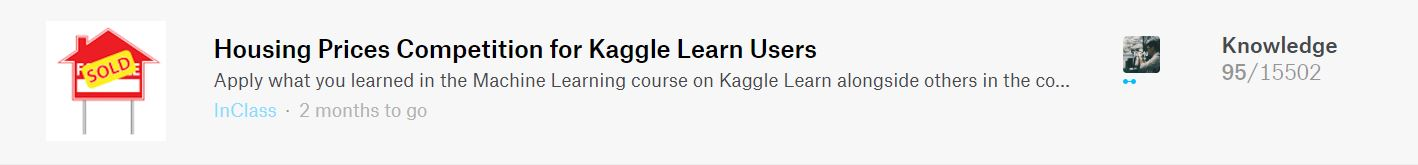Import libraries

In :
import numpy as np
import pandas as pd
import matplotlib.pyplot as plt
import seaborn as sns

import warnings
warnings.simplefilter(action='ignore', category=FutureWarning)

%matplotlib inline
sns.set_style('darkgrid')


In :
# Load data

# Seperate features and target variable
X_train = train_data.drop(['SalePrice'], axis=1)
y = train_data.SalePrice

# Concatenate train and test data
X = pd.concat([X_train, test_data], axis=0)


Describe data

In :
print("Train data's size: ", X_train.shape)
print("Test data's size: ", test_data.shape)

Train data's size:  (1460, 79)
Test data's size:  (1459, 79)

In :
numCols = list(X_train.select_dtypes(exclude='object').columns)
print(f"There are {len(numCols)} numerical features:\n", numCols)

There are 36 numerical features:
['MSSubClass', 'LotFrontage', 'LotArea', 'OverallQual', 'OverallCond', 'YearBuilt', 'YearRemodAdd', 'MasVnrArea', 'BsmtFinSF1', 'BsmtFinSF2', 'BsmtUnfSF', 'TotalBsmtSF', '1stFlrSF', '2ndFlrSF', 'LowQualFinSF', 'GrLivArea', 'BsmtFullBath', 'BsmtHalfBath', 'FullBath', 'HalfBath', 'BedroomAbvGr', 'KitchenAbvGr', 'TotRmsAbvGrd', 'Fireplaces', 'GarageYrBlt', 'GarageCars', 'GarageArea', 'WoodDeckSF', 'OpenPorchSF', 'EnclosedPorch', '3SsnPorch', 'ScreenPorch', 'PoolArea', 'MiscVal', 'MoSold', 'YrSold']

In :
catCols = list(X_train.select_dtypes(include='object').columns)
print(f"There are {len(catCols)} numerical features:\n", catCols)

There are 43 numerical features:
['MSZoning', 'Street', 'Alley', 'LotShape', 'LandContour', 'Utilities', 'LotConfig', 'LandSlope', 'Neighborhood', 'Condition1', 'Condition2', 'BldgType', 'HouseStyle', 'RoofStyle', 'RoofMatl', 'Exterior1st', 'Exterior2nd', 'MasVnrType', 'ExterQual', 'ExterCond', 'Foundation', 'BsmtQual', 'BsmtCond', 'BsmtExposure', 'BsmtFinType1', 'BsmtFinType2', 'Heating', 'HeatingQC', 'CentralAir', 'Electrical', 'KitchenQual', 'Functional', 'FireplaceQu', 'GarageType', 'GarageFinish', 'GarageQual', 'GarageCond', 'PavedDrive', 'PoolQC', 'Fence', 'MiscFeature', 'SaleType', 'SaleCondition']


Data dictionary can be found here.

## 2. Exploratory Data Analysis¶

### 2.1. Sale Price¶

In :
plt.figure(figsize=(8,6))
sns.distplot(y)
title = plt.title("House Price Distribution")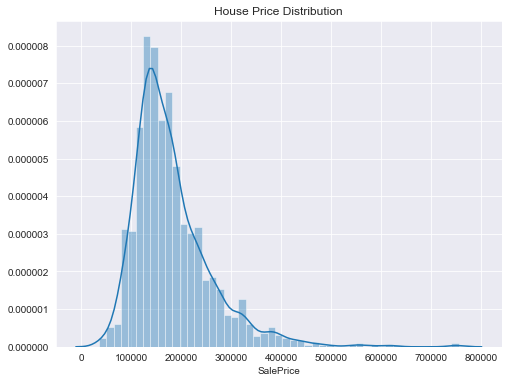The distribution of SalePrice is right-skewed. Let's check its Skewness and Kurtosis statistics.

In :
print(f"""Skewness: {y.skew()}
Kurtosis: {y.kurt()}""")

Skewness: 1.8828757597682129
Kurtosis: 6.536281860064529


### 2.2. Numerical Features¶

Top 10 numerical variables highly correlated with SalePrice:

In :
corr_mat = train_data.corr().SalePrice.sort_values(ascending=False)

Out:
SalePrice       1.000000
OverallQual     0.790982
GrLivArea       0.708624
GarageCars      0.640409
GarageArea      0.623431
TotalBsmtSF     0.613581
1stFlrSF        0.605852
FullBath        0.560664
TotRmsAbvGrd    0.533723
YearBuilt       0.522897
Name: SalePrice, dtype: float64

What are the top 10 features selected by Recursive Feature Elimination?

In :
from sklearn.feature_selection import RFE
from sklearn.linear_model import LinearRegression

estimator = LinearRegression()
rfe = RFE(estimator, n_features_to_select=10, step=1)
selector = rfe.fit(X_train.fillna(0).select_dtypes(exclude='object'), y)
selectedFeatures = list(
X.select_dtypes(exclude='object').columns[selector.support_])
selectedFeatures

Out:
['OverallQual',
'BsmtFullBath',
'BsmtHalfBath',
'FullBath',
'HalfBath',
'BedroomAbvGr',
'KitchenAbvGr',
'TotRmsAbvGrd',
'Fireplaces',
'GarageCars']

According to above analyses, Overall Quality, Living Area, Number of Full Baths, Size of Garage and Year Built are some of the most important features in determining house price. Let's take a closer look at them.

Overall Quality

Overall quality is the most important feature in both analyses. It is clear that higher quality makes the house more expensive.

In :
plt.figure(figsize=(8, 6))
sns.boxplot(x='OverallQual', y='SalePrice', data=train_data, palette='GnBu')
title = plt.title('House Price by Overall Quality')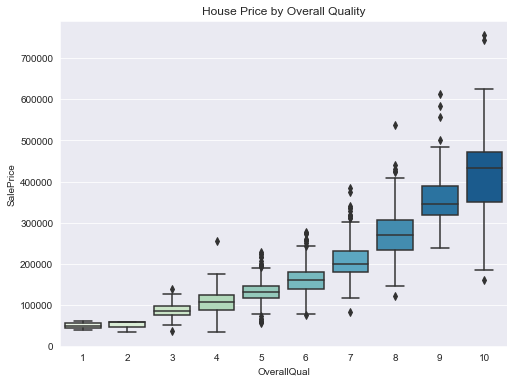Living Area

Living area has a linear relationship with house price. In the scatter plot below, we can clearly see some outliers in the data, especially the two houses in the lower-right corner with living area greater than 4000 sqft and price lower than $200,000. In : def plotCorrelation(variables): """ 1. Print correlation of two variables 2. Create jointplot of two variables """ # Print correlation print("Correlation: ", train_data[[variables, variables]].corr().iloc[1, 0]) # Create jointplot plt.figure(figsize=(6, 6)) sns.jointplot(train_data[(variables)], train_data[(variables)], kind='reg', height=7, scatter_kws={'s': 10}, marginal_kws={'kde': True}) plotCorrelation(['GrLivArea', 'SalePrice'])  Correlation: 0.7086244776126511  <Figure size 432x432 with 0 Axes>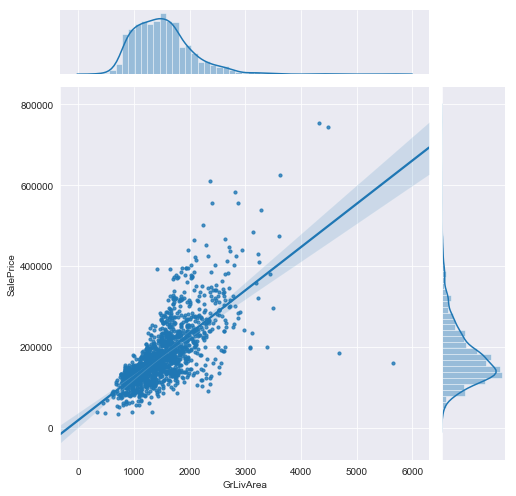GarageCars Interestingly, houses with garage which can hold 4 cars are cheaper than houses with 3-car garage. In : plt.figure(figsize=(8, 6)) sns.boxplot(x='GarageCars', y='SalePrice', data=train_data, palette='GnBu') title = plt.title('House Price by Garage Size')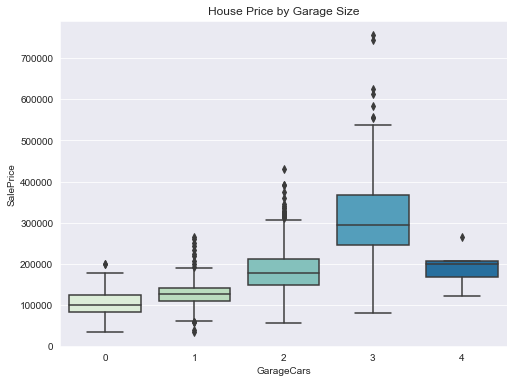Year Built The age of the house also plays an important role in its price. Newer houses have higher average prices. There are several houses built before 1900 having a high price. In : plt.figure(figsize=(15, 6)) sns.scatterplot(x='YearBuilt', y='SalePrice', data=train_data) title = plt.title('House Price by Year Built')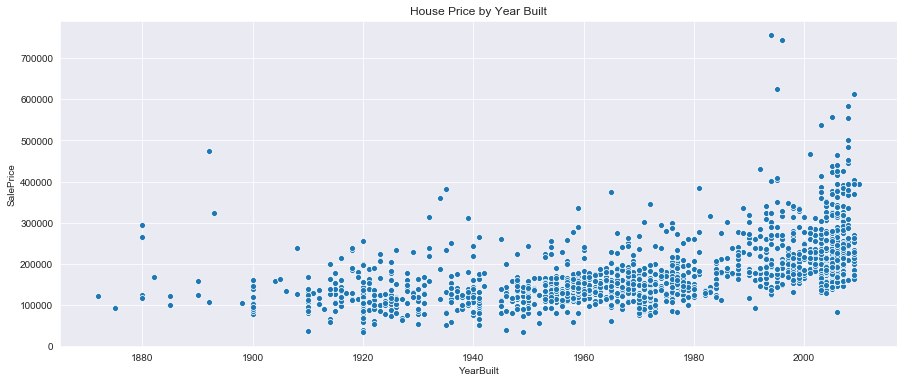### 2.3. Categorical Variables¶ Using ANOVA, I have identified 15 categorical features having p-values lower than 0.01: In : sigCatCols = [ 'Street', 'LandContour', 'LotConfig', 'LandSlope', 'Neighborhood', 'Condition1', 'Condition2', 'RoofMatl', 'ExterQual', 'BsmtQual', 'BsmtExposure', 'KitchenQual', 'Functional', 'GarageQual', 'PoolQC' ]  Let's explore some of them. In : def visualizeCatFeature(feature): """ Visualize the relationship between SalePrice and categorical feature using box plots """ # Descending order of levels sorted by median SalePrice featOrder = train_data.groupby( [feature]).median().SalePrice.sort_values(ascending=False).index # Create box plot sns.boxplot(x=feature, y='SalePrice', data=train_data, order=featOrder, palette='GnBu_r')  Neighborhood There is a big difference in house prices among neighborhood in Ames. The top 3 expensive neighborhoods are NridgHt, NoRidge and StoneBr with median house prices of approximately$300,000, three times as high as the median of the 3 cheapest neighborhoods, which are BrDale, DOTRR and MeadowV.

In :
plt.figure(figsize=(12, 6))
visualizeCatFeature('Neighborhood')
title = plt.title('House Price by Neighborhood')
tick = plt.xticks(rotation=45)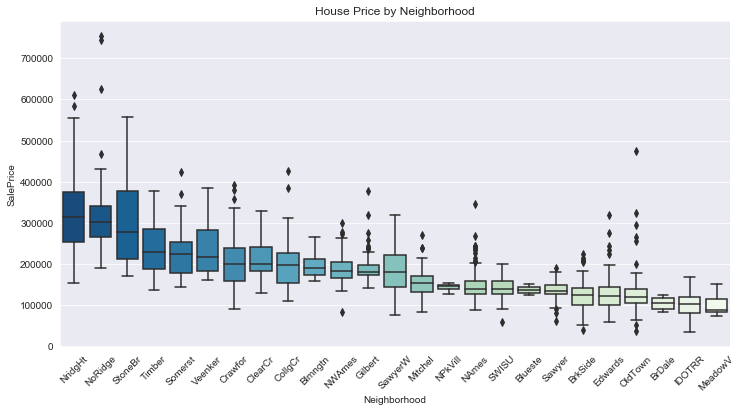Roof Material

Houses using Wood Shingles roof are the most expensive with price ranging from \$300,000 to \\$450,000. There are also a lot of expensive houses using Standard Composite Shingle roof.

In :
plt.figure(figsize=(8, 6))
visualizeCatFeature('RoofMatl')
title = plt.title('House Price by Roof Material')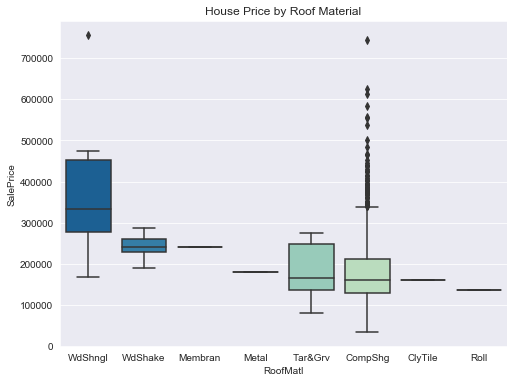Kitchen Quality

Kitchen Quality is another important feature to predict house price. There is a very big difference in price between houses with different kitchen quality. For example, the average price difference between a house with a good kitchen and one with an excellent kitchen is about $120,000. In : plt.figure(figsize=(8, 6)) visualizeCatFeature('KitchenQual') title = plt.title('House Price by Kitchen Quality')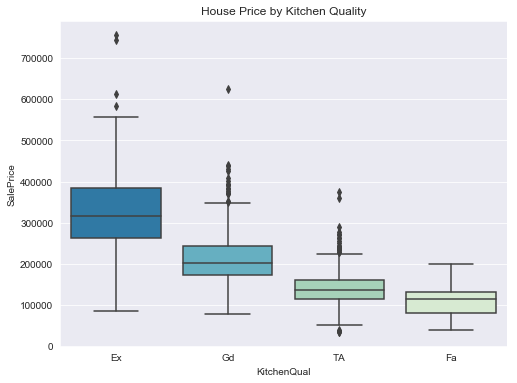### 2.4. Correlations¶ In : # Create correlation matrix from train data excluding SalePrice corr_mat = train_data.iloc[:, :-1].corr() # Select correlations greater than 0.5 high_corr_mat = corr_mat[abs(corr_mat) >= 0.5] # Plot correlation heatmap plt.figure(figsize=(15, 10)) sns.heatmap(high_corr_mat, annot=True, fmt='.1f', cmap='GnBu', vmin=0.5, vmax=1) title = plt.title('Correlation Heatmap')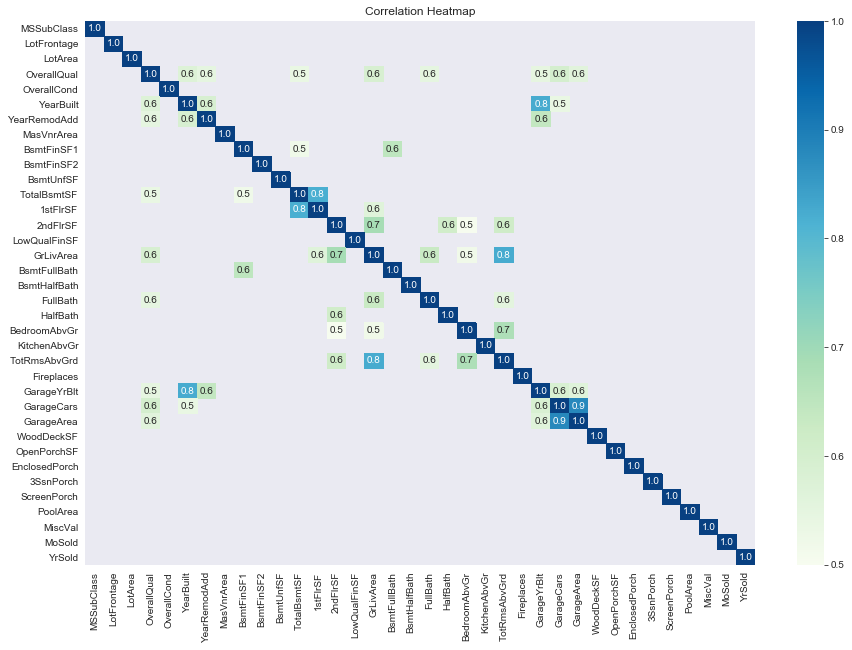There is multicollinearity in our training data. Below features are highly correlated: - GarageCars and GarageArea - GarageYrBlt and YearBuilt - 1stFlrSF and TotalBsmtSF - GrLivArea and TotRmsAbvGrd  Multicolliniearity has a negative impact on our prediction models and makes standard errors of our estimates increase. Therefore, for each pair of highly correlated features, I will remove a feature that has a lower correlation with SalePrice. ### 2.5. Missing Values¶ Most machine learning algorithms give an error when we train them on data with missing values. Therefore, it's important to identify them before deciding how to handle them (drop features or impute missing value). In : missing_data_count = X.isnull().sum() missing_data_percent = X.isnull().sum() / len(X) * 100 missing_data = pd.DataFrame({ 'Count': missing_data_count, 'Percent': missing_data_percent }) missing_data = missing_data[missing_data.Count > 0] missing_data.sort_values(by='Count', ascending=False, inplace=True) print(f"There are {missing_data.shape} features having missing data.\n") print("Top 10 missing value features:") missing_data.head(10)  There are 34 features having missing data. Top 10 missing value features:  Out: Count Percent PoolQC 2909 99.657417 MiscFeature 2814 96.402878 Alley 2721 93.216855 Fence 2348 80.438506 FireplaceQu 1420 48.646797 LotFrontage 486 16.649538 GarageFinish 159 5.447071 GarageQual 159 5.447071 GarageCond 159 5.447071 GarageYrBlt 159 5.447071 In : plt.figure(figsize=(12, 6)) sns.barplot(y=missing_data.head(18).index, x=missing_data.head(18).Count, palette='GnBu_r') title = plt.title("Missing Values")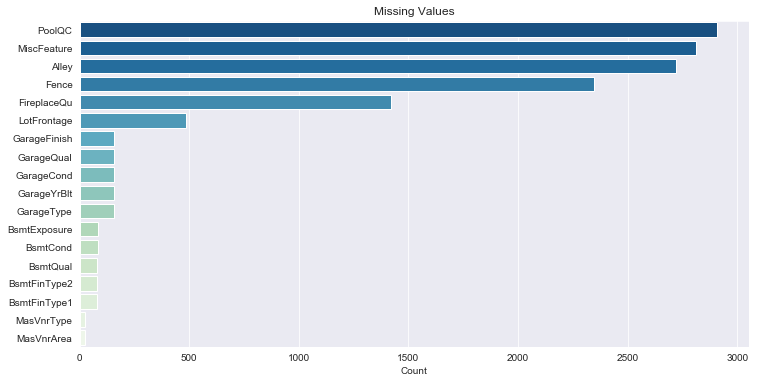With some basic understandings of the data set and features, let's move to data preprocessing and modeling steps. ## 3. Data Preprocessing and Feature Engineering¶ ### 3.1. Missing Values¶ In : missing_data_count = X.isnull().sum() missing_data_percent = X.isnull().sum() / len(X) * 100 missing_data = pd.DataFrame({ 'Count': missing_data_count, 'Percent': missing_data_percent }) missing_data = missing_data[missing_data.Count > 0] missing_data.sort_values(by='Count', ascending=False, inplace=True)  In : missing_data.head(10)  Out: Count Percent PoolQC 2909 99.657417 MiscFeature 2814 96.402878 Alley 2721 93.216855 Fence 2348 80.438506 FireplaceQu 1420 48.646797 LotFrontage 486 16.649538 GarageFinish 159 5.447071 GarageQual 159 5.447071 GarageCond 159 5.447071 GarageYrBlt 159 5.447071 There are 34 features that have missing values. I will divide them into three groups based on the data description: • Group 1 - Categorical variables where NA means no feature: PoolQC, MiscFeature, Alley, Fence, FireplaceQu, GarageType, GarageFinish, GarageQual, GarageCond, BsmtQual, BsmtCond, BsmtExposure, BsmtFinType1, BsmtFinType2, MasVnrType (15 variables) For this group I will impute NA with 'None'. • Group 2 - Numerical variables where NA means no feature: GarageArea, GarageCars, BsmtFinSF1, BsmtFinSF2, BsmtUnfSF, TotalBsmtSF, BsmtFullBath, BsmtHalfBath, MasVnrArea (10 variables) For this group I will impute NA with 0. • Group 3 - Other variables: Functional, MSZoning, Electrical, KitchenQual, Exterior1st, Exterior2nd, SaleType, Utilities, LotFrontage, GarageYrBlt (9 variables) • I will impute Functional, MSZoning, Electrical, KitchenQual, Exterior1st, Exterior2nd, SaleType, Utilities with their modes, • impute LotFrontage with its mean, • impute GarageYrBlt with YearBuilt. In : from sklearn.impute import SimpleImputer # Group 1: group_1 = [ 'PoolQC', 'MiscFeature', 'Alley', 'Fence', 'FireplaceQu', 'GarageType', 'GarageFinish', 'GarageQual', 'GarageCond', 'BsmtQual', 'BsmtCond', 'BsmtExposure', 'BsmtFinType1', 'BsmtFinType2', 'MasVnrType' ] X[group_1] = X[group_1].fillna("None") # Group 2: group_2 = [ 'GarageArea', 'GarageCars', 'BsmtFinSF1', 'BsmtFinSF2', 'BsmtUnfSF', 'TotalBsmtSF', 'BsmtFullBath', 'BsmtHalfBath', 'MasVnrArea' ] X[group_2] = X[group_2].fillna(0) # Group 3: group_3a = [ 'Functional', 'MSZoning', 'Electrical', 'KitchenQual', 'Exterior1st', 'Exterior2nd', 'SaleType', 'Utilities' ] imputer = SimpleImputer(strategy='most_frequent') X[group_3a] = pd.DataFrame(imputer.fit_transform(X[group_3a]), index=X.index) X.LotFrontage = X.LotFrontage.fillna(X.LotFrontage.mean()) X.GarageYrBlt = X.GarageYrBlt.fillna(X.YearBuilt)  Let's check whether there is any missing value left: In : sum(X.isnull().sum())  Out: 0 Great! All missing values have been handled. ### 3.2. Outliers¶ Because regression models are very sensitive to outlier, we need to be aware of them. Let's examine outliers with a scatter plot. In : sns.set_style('darkgrid') plt.figure(figsize=(8, 6)) sns.scatterplot(x='GrLivArea', y='SalePrice', data=train_data) title = plt.title('House Price vs. Living Area')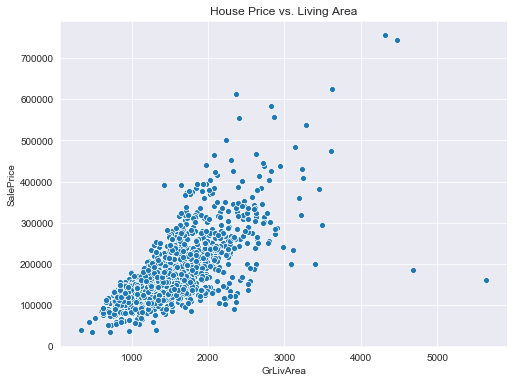There are two observations lying separately from the rest. They have large living area but low price. They are the outliers that we are looking for. I will delete them from the training set. In : outlier_index = train_data[(train_data.GrLivArea > 4000) & (train_data.SalePrice < 200000)].index X.drop(outlier_index, axis=0, inplace=True) y.drop(outlier_index, axis=0, inplace=True)  ### 3.3. Feature Engineering¶ #### 3.3.1. Create New Variables¶ In this step I will create new features from weaker features in the training data. For example, the surface area of each floor has low correlation with house price; however, when we sum them up, the relationship becomes much stronger. In fact, TotalSqFeet becomes the strongest feature in the dataset. The new features I will create are total square feet, total number of bathrooms, age of the house, whether the house was remodeled, and whether the house was sold in the same year it was built. In : X['totalSqFeet'] = X['TotalBsmtSF'] + X['1stFlrSF'] + X['2ndFlrSF'] X['totalBathroom'] = X.FullBath + X.BsmtFullBath + 0.5 * (X.HalfBath + X.BsmtHalfBath) X['houseAge'] = X.YrSold - X.YearBuilt X['reModeled'] = np.where(X.YearRemodAdd == X.YearBuilt, 0, 1) X['isNew'] = np.where(X.YrSold == X.YearBuilt, 1, 0)  #### 3.3.2. Label Encoding¶ Ordinal categorical features are label encoded. In : from sklearn.preprocessing import LabelEncoder # Ordinal categorical columns label_encoding_cols = [ "Alley", "BsmtCond", "BsmtExposure", "BsmtFinType1", "BsmtFinType2", "BsmtQual", "ExterCond", "ExterQual", "FireplaceQu", "Functional", "GarageCond", "GarageQual", "HeatingQC", "KitchenQual", "LandSlope", "LotShape", "PavedDrive", "PoolQC", "Street", "Utilities" ] # Apply Label Encoder label_encoder = LabelEncoder() for col in label_encoding_cols: X[col] = label_encoder.fit_transform(X[col])  #### 3.3.3. Transform Numerical Variables to Categorical Variables¶ Because I have calculated age of houses, YearBuilt is no longer needed. However, YrSold could have a large impact on house price (e.g. In economic crisis years, house price could be lower). Therefore, I will transform it into categorical variables. Like YrSold, some numerical variables don't have any ordinal meaning (e.g. MoSold, MSSubClass). I will transform them into categorical variables. In : to_factor_cols = ['YrSold', 'MoSold', 'MSSubClass'] for col in to_factor_cols: X[col] = X[col].apply(str)  ### 3.4. Skewness and Normalizing Variables¶ Normal distribution is one of the assumption that linear regression relies on. Therefore, transfoming skewed data will help our models perform better. First, let's examine the target variable SalePrice with Distribution plot and Quantile-Quantile plot. Target variable In : from scipy import stats from scipy.stats import norm  In : def normality_plot(X): """ 1. Draw distribution plot with normal distribution fitted curve 2. Draw Quantile-Quantile plot """ fig, axes = plt.subplots(1, 2, figsize=(10, 5)) sns.distplot(X, fit=norm, ax=axes) axes.set_title('Distribution Plot') axes = stats.probplot((X), plot=plt) plt.tight_layout()  In : normality_plot(y)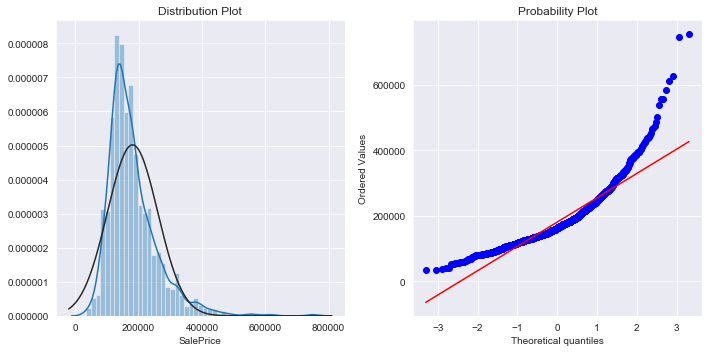One of the methods to normalize right-skewed data is using log transformation because big values will be pulled to the center. However, log(0) is Nan, so I will use log(1+X) to fix skewness instead. In : y = np.log(1 + y)  And this is SalePrice after log transformation. The sknewness has been fixed. In : normality_plot(y)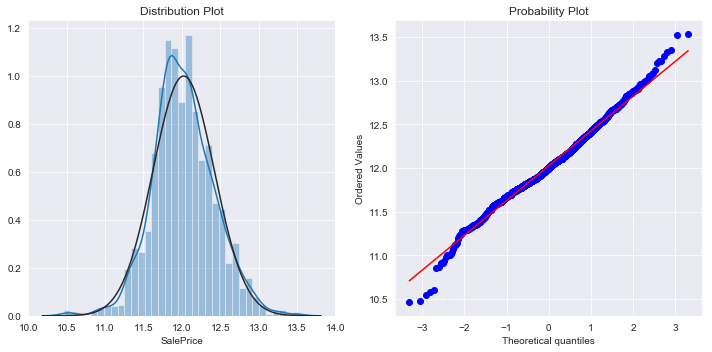In the next step I will examine skewness in the rest of numerical variables and use log transformation to fix them, Fixing skewness in other numerical variables If skewness is less than -1 or greater than 1, the distribution is highly skewed. If skewness is between -1 and -0.5 or between 0.5 and 1, the distribution is moderately skewed. If skewness is between -0.5 and 0.5, the distribution is approximately symmetric. Below are skewed features in our original train data. In : skewness = train_data.skew().sort_values(ascending=False) skewness[abs(skewness) > 0.75]  Out: MiscVal 24.476794 PoolArea 14.828374 LotArea 12.207688 3SsnPorch 10.304342 LowQualFinSF 9.011341 KitchenAbvGr 4.488397 BsmtFinSF2 4.255261 ScreenPorch 4.122214 BsmtHalfBath 4.103403 EnclosedPorch 3.089872 MasVnrArea 2.669084 OpenPorchSF 2.364342 LotFrontage 2.163569 SalePrice 1.882876 BsmtFinSF1 1.685503 WoodDeckSF 1.541376 TotalBsmtSF 1.524255 MSSubClass 1.407657 1stFlrSF 1.376757 GrLivArea 1.366560 BsmtUnfSF 0.920268 2ndFlrSF 0.813030 dtype: float64 Let's check normality of GrLivArea: In : normality_plot(X.GrLivArea)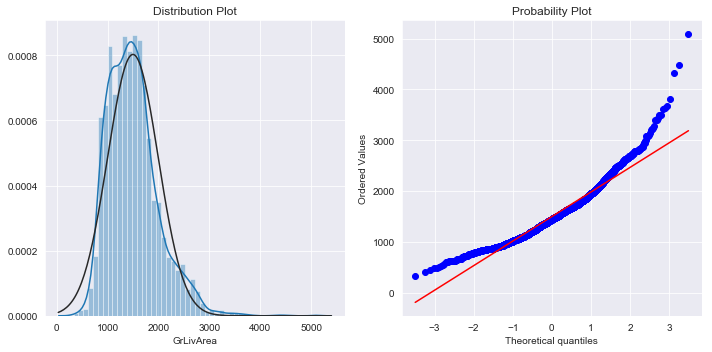In : # List of skewed columns skewed_cols = list(skewness[abs(skewness) > 0.5].index) # Remove 'MSSubClass' and 'SalePrice' skewed_cols = [ col for col in skewed_cols if col not in ['MSSubClass', 'SalePrice'] ] # Log-transform skewed columns for col in skewed_cols: X[col] = np.log(1 + X[col])  Below is normality of GrLivArea after log-transformation. Skewness has been fixed. In : normality_plot(X.GrLivArea)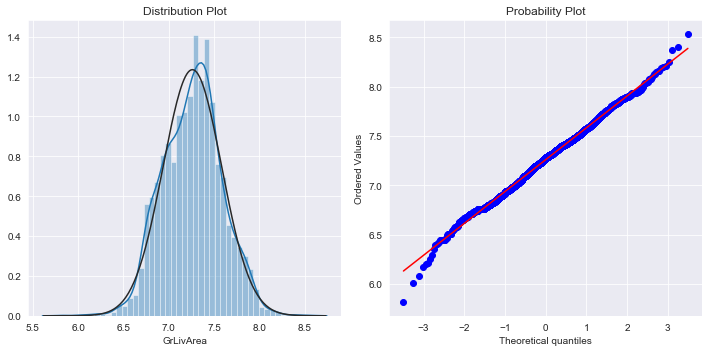### 3.5. Feature Scaling¶ Except for Decision Tree and Random Forest, it is highly recommended to standardize the data set before running machine learning algorithms since optimization methods and gradient descent run and converge faster on similarly scaled features. However, outliers can often influence the sample mean and standard deviation in a negative way, and models like Lasso and Elastic Net are very sensitive to outliers. In such cases, the median and the interquartile range often give better results. I will use RobustScaler to transform the training data. In : from sklearn.preprocessing import RobustScaler numerical_cols = list(X.select_dtypes(exclude=['object']).columns) scaler = RobustScaler() X[numerical_cols] = scaler.fit_transform(X[numerical_cols])  ### 3.6. One-hot Encoding¶ In : X = pd.get_dummies(X, drop_first=True) print("X.shape:", X.shape)  X.shape: (2917, 237)  After preprocessing the train and test data, I split them again to perform modeling. In : ntest = len(test_data) X_train = X.iloc[:-ntest, :] X_test = X.iloc[-ntest:, :] print("X_train.shape:", X_train.shape) print("X_test.shape:", X_test.shape)  X_train.shape: (1458, 237) X_test.shape: (1459, 237)  ## 4. Modeling¶ In model evaluation, it's a common practice to split the entire training data into 2 sets of data (train and test). However, a model may work very well on a set of test data but have a poor performance on other sets of unseen data. A solution to this problem is a procedure called cross-validation (CV). In the example below, under the basic approach, called k-fold CV, the training set is split into 5 smaller sets. Then, for each fold, a model is trained using the other 4 folds and evaluated on the remaining fold. The performance measure reported by k-fold cross-validation is then the average of the values computed in the loop.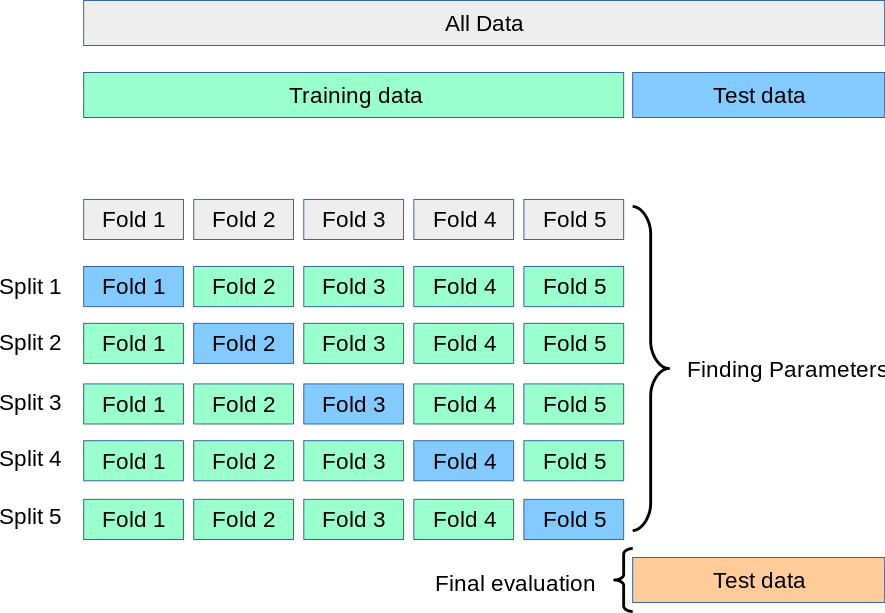I will write a function to get the Root Mean Squared Logarithmic Error (RMSLE) for my models using cross-validation. There is one note here: because I have transformed the target variable to log(1+y) , the Mean Squared Error for log(1+y) is the Mean Squared Logarithmic Error for SalePrice. In : from sklearn.model_selection import KFold, cross_val_score n_folds = 5 def getRMSLE(model): """ Return the average RMSLE over all folds of training data. """ # Set KFold to shuffle data before the split kf = KFold(n_folds, shuffle=True, random_state=42) # Get RMSLE score rmse = np.sqrt(-cross_val_score( model, X_train, y, scoring="neg_mean_squared_error", cv=kf)) return rmse.mean()  ### 4.1. Regularized Regressions¶ In : from sklearn.linear_model import Ridge, Lasso  #### 4.1.1. Ridge Regression¶ In the regularized linear regression (Ridge), we try to minimize: $$J(\theta) = \frac{1}{2m} \left( \sum_{i=1}^m \left( h_\theta\left( x^{(i)} \right) - y^{(i)} \right)^2 \right) + \frac{\lambda}{2m} \left( \sum_{j=1}^n \theta_j^2 \right)$$ where$\lambda$is a regularization parameter which controls the degree of regularization (thus, help preventing overfitting). The regularization term puts a penalty on the overall cost J. As the magnitudes of the model parameters$\theta_j$increase, the penalty increases as well. I will find the$\lambda$that gives me the smallest RMSLE from cross-validation: In : lambda_list = list(np.linspace(20, 25, 101)) rmsle_ridge = [getRMSLE(Ridge(alpha=lambda_)) for lambda_ in lambda_list] rmsle_ridge = pd.Series(rmsle_ridge, index=lambda_list) rmsle_ridge.plot(title="RMSLE by lambda") plt.xlabel("Lambda") plt.ylabel("RMSLE") print("Best lambda:", rmsle_ridge.idxmin()) print("RMSLE:", rmsle_ridge.min())  Best lambda: 22.9 RMSLE: 0.11409306668450885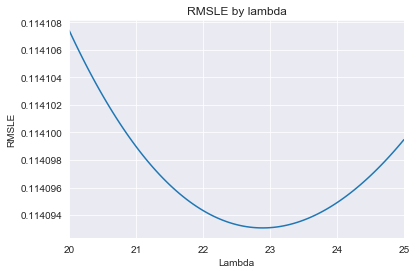In : ridge = Ridge(alpha=22.9)  #### 4.1.2. Lasso Regression¶ Lasso Regression is very similar to Ridge regression. One difference is that in the regularization term, instead of using sum of squared of$\theta$, we use sum of absolute value of$\theta\$:

$$J(\theta) = \frac{1}{2m} \left( \sum_{i=1}^m \left( h_\theta\left( x^{(i)} \right) - y^{(i)} \right)^2 \right) + \frac{\lambda}{2m} \left( \sum_{j=1}^n |\theta_j| \right)$$

Another big difference is that Ridge Regresion can only shrink parameters close to zero while Lasso Regression can shrink some parameters all the way to 0. Therefore, we can use Lasso Regression to perform feature selection and regression.

With the same method above, the best lambda_ for my Lasso model is 0.00065.

In :
lambda_list = list(np.linspace(0.0006, 0.0007, 11))
rmsle_lasso = [
getRMSLE(Lasso(alpha=lambda_, max_iter=100000)) for lambda_ in lambda_list
]
rmsle_lasso = pd.Series(rmsle_lasso, index=lambda_list)

rmsle_lasso.plot(title="RMSLE by lambda")
plt.xlabel("Lambda")
plt.ylabel("RMSLE")

print("Best lambda:", rmsle_lasso.idxmin())
print("RMSLE:", rmsle_lasso.min())

Best lambda: 0.00065
RMSLE: 0.11335701578061286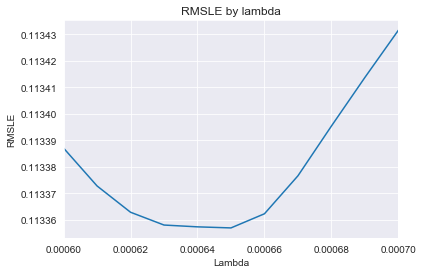In :
lasso = Lasso(alpha=0.00065, max_iter=